Previous Page | Next Page

 The GENMOD Procedure

## Example 37.10 Bayesian Analysis of a Poisson Regression Model

This example illustrates a Bayesian analysis of a log-linear Poisson regression model. Consider the following data on patients from clinical trials. The data set is a subset of the data described in Ibrahim, Chen, and Lipsitz (1999).

```data Liver;
input X1-X6 Y;
datalines;
19.1358    50.0110     51.000      0        0       1        3
23.5970    18.4959      3.429      0        0       1        9
20.0474    56.7699      3.429      1        1       0        6
28.0277    59.7836      4.000      0        0       1        6
28.6851    74.1589      5.714      1        0       1        1
18.8092    31.0630      2.286      0        1       1       61
28.7201    52.9178     37.286      1        0       1        6
21.3669    61.6603     54.143      0        1       1        6
23.7332    42.2904      0.571      1        0       1       21

... more lines ...

19.1327    65.3425      2.571      1        0       0       1
17.3010    51.4493      4.429      1        0       0       6
;
run;
```

The primary interest is in prediction of the number of cancerous liver nodes when a patient enters the trials, by using six other baseline characteristics. The number of nodes is modeled by a Poisson regression model with the six baseline characteristics as covariates. The response and regression variables are as follows:

 Y Number of Cancerous Liver Nodes X1 Body Mass Index X2 Age, in Years X3 Time Since Diagnosis of Disease, in Weeks X4 Two Biochemical Markers (each classified as normal=1 or abnormal=0) X5 Anti Hepatitis B Antigen X6 Associated Jaundice (yes=1, no=0)

Two analyses are performed using PROC GENMOD. The first analysis uses noninformative normal prior distributions, and the second analysis uses an informative normal prior for one of the regression parameters.

In the following BAYES statement, COEFFPRIOR=NORMAL specifies a noninformative independent normal prior distribution with zero mean and variance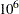for each parameter.

The initial analysis is performed using PROC GENMOD to obtain Bayesian estimates of the regression coefficients by using the following SAS statements:

```ods graphics ON;
proc genmod data=Liver;
model Y = X1-X6 / dist=Poisson link=log;
bayes seed=1 coeffprior=normal;
run;
```

Maximum likelihood estimates of the model parameters are computed by default. These are shown in the "Analysis of Maximum Likelihood Parameter Estimates" table in Output 37.10.1.

Output 37.10.1 Maximum Likelihood Parameter Estimates
The GENMOD Procedure

Bayesian Analysis

Analysis Of Maximum Likelihood Parameter Estimates
Parameter DF Estimate Standard Error Wald 95% Confidence Limits
Intercept 1 2.4508 0.2284 2.0032 2.8984
X1 1 -0.0044 0.0080 -0.0201 0.0114
X2 1 -0.0135 0.0024 -0.0181 -0.0088
X3 1 -0.0029 0.0022 -0.0072 0.0014
X4 1 -0.2715 0.0795 -0.4272 -0.1157
X5 1 0.3215 0.0832 0.1585 0.4845
X6 1 0.2077 0.0827 0.0456 0.3698
Scale 0 1.0000 0.0000 1.0000 1.0000

 Note: The scale parameter was held fixed.

Noninformative independent normal prior distributions with zero means and variances ofwere used in the initial analysis. These are shown in Output 37.10.2.

Output 37.10.2 Regression Coefficient Priors
The GENMOD Procedure

Bayesian Analysis

Independent Normal Prior for Regression
Coefficients
Parameter Mean Precision
Intercept 0 1E-6
X1 0 1E-6
X2 0 1E-6
X3 0 1E-6
X4 0 1E-6
X5 0 1E-6
X6 0 1E-6

Initial values for the Markov chain are listed in the "Initial Values and Seeds" table in Output 37.10.3. The random number seed is also listed so that you can reproduce the analysis. Since no seed was specified, the seed shown was derived from the time of day.
Output 37.10.3 MCMC Initial Values and Seeds
Initial Values of the Chain
Chain Seed Intercept X1 X2 X3 X4 X5 X6
1 1 2.450813 -0.00435 -0.01347 -0.00291 -0.27149 0.321507 0.207713

Summary statistics for the posterior sample are displayed in the "Fit Statistics," "Descriptive Statistics for the Posterior Sample," "Interval Statistics for the Posterior Sample," and "Posterior Correlation Matrix" tables in Output 37.10.4, Output 37.10.5, Output 37.10.6, and Output 37.10.7, respectively. Since noninformative prior distributions for the regression coefficients were used, the mean and standard deviations of the posterior distributions for the model parameters are close to the maximum likelihood estimates and standard errors.

Output 37.10.4 Fit Statistics
Fit Statistics
DIC (smaller is better) 829.729
pD (effective number of parameters) 6.966

Output 37.10.5 Descriptive Statistics
The GENMOD Procedure

Bayesian Analysis

Posterior Summaries
Parameter N Mean Standard
Deviation
Percentiles
25% 50% 75%
Intercept 10000 2.4520 0.2268 2.2997 2.4521 2.6053
X1 10000 -0.00473 0.00801 -0.0100 -0.00465 0.000759
X2 10000 -0.0134 0.00236 -0.0150 -0.0134 -0.0118
X3 10000 -0.00309 0.00220 -0.00455 -0.00305 -0.00158
X4 10000 -0.2705 0.0792 -0.3241 -0.2697 -0.2172
X5 10000 0.3193 0.0834 0.2629 0.3180 0.3762
X6 10000 0.2095 0.0834 0.1538 0.2086 0.2653

Output 37.10.6 Interval Statistics
Posterior Intervals
Parameter Alpha Equal-Tail Interval HPD Interval
Intercept 0.050 2.0169 2.9056 2.0069 2.8923
X1 0.050 -0.0210 0.0106 -0.0212 0.0103
X2 0.050 -0.0181 -0.00878 -0.0181 -0.00885
X3 0.050 -0.00757 0.00109 -0.00764 0.000989
X4 0.050 -0.4250 -0.1132 -0.4232 -0.1119
X5 0.050 0.1552 0.4821 0.1647 0.4905
X6 0.050 0.0477 0.3749 0.0490 0.3758

Output 37.10.7 Posterior Sample Correlation Matrix
Posterior Correlation Matrix
Parameter Intercept X1 X2 X3 X4 X5 X6
Intercept 1.000 -0.705 -0.430 -0.046 -0.225 -0.180 -0.415
X1 -0.705 1.000 -0.211 -0.013 -0.068 0.067 0.128
X2 -0.430 -0.211 1.000 -0.006 0.070 0.057 0.118
X3 -0.046 -0.013 -0.006 1.000 0.016 -0.055 -0.089
X4 -0.225 -0.068 0.070 0.016 1.000 -0.011 0.089
X5 -0.180 0.067 0.057 -0.055 -0.011 1.000 -0.042
X6 -0.415 0.128 0.118 -0.089 0.089 -0.042 1.000

Posterior sample autocorrelations for each model parameter are shown in Output 37.10.8. The autocorrelation after 10 lags is negligible for all parameters, indicating good mixing in the Markov chain.

Output 37.10.8 Posterior Sample Autocorrelations
The GENMOD Procedure

Bayesian Analysis

Posterior Autocorrelations
Parameter Lag 1 Lag 5 Lag 10 Lag 50
Intercept 0.0551 -0.0134 -0.0101 0.0012
X1 0.0894 -0.0054 -0.0080 0.0019
X2 0.1197 -0.0170 0.0061 0.0006
X3 0.0324 -0.0036 -0.0033 -0.0160
X4 0.0309 0.0056 0.0053 0.0115
X5 0.0402 0.0015 -0.0111 0.0123
X6 0.0696 -0.0047 -0.0024 0.0006

The p-values for the Geweke test statistics shown in Output 37.10.9 all indicate convergence of the MCMC. See the section Assessing Markov Chain Convergence for more information about convergence diagnostics and their interpretation.

Output 37.10.9 Geweke Diagnostic Statistics
Geweke Diagnostics
Parameter z Pr > |z|
Intercept 0.9855 0.3244
X1 -1.0835 0.2786
X2 -0.3847 0.7005
X3 0.6715 0.5019
X4 0.1328 0.8943
X5 1.0698 0.2847
X6 -0.1647 0.8692

The effective sample sizes for each parameter are shown in Output 37.10.10.

Output 37.10.10 Effective Sample Sizes
Effective Sample Sizes
Parameter ESS Autocorrelation
Time
Efficiency
Intercept 9245.8 1.0816 0.9246
X1 8179.5 1.2226 0.8179
X2 8067.8 1.2395 0.8068
X3 9390.6 1.0649 0.9391
X4 9157.6 1.0920 0.9158
X5 9665.2 1.0346 0.9665
X6 8778.7 1.1391 0.8779

Trace, autocorrelation, and density plots for the seven model parameters are shown in Output 37.10.11 through Output 37.10.17. All indicate satisfactory convergence of the Markov chain.

Output 37.10.11 Diagnostic Plots for Intercept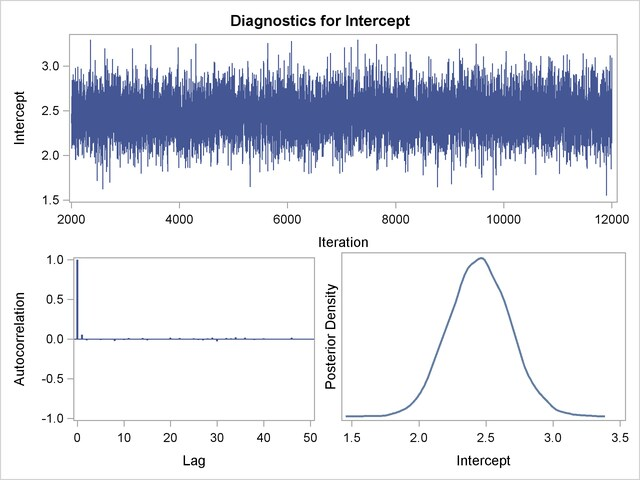Output 37.10.12 Diagnostic Plots for X1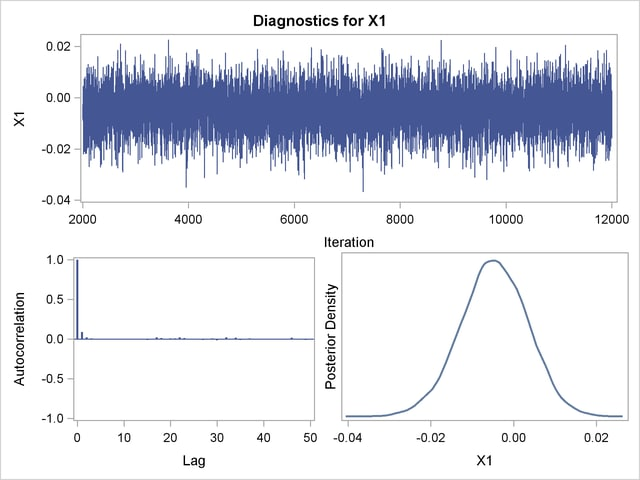Output 37.10.13 Diagnostic Plots for X2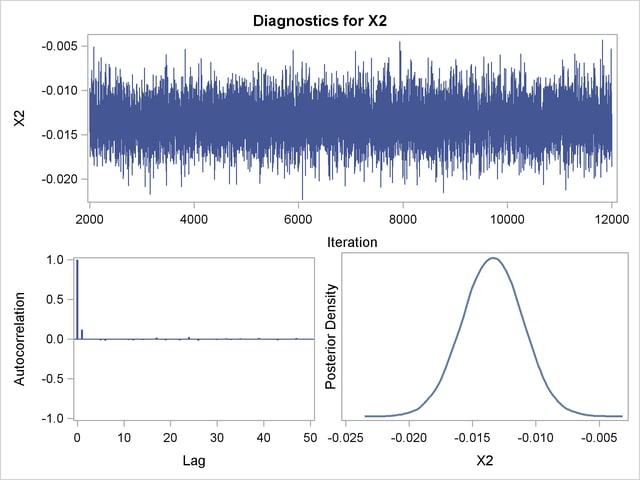Output 37.10.14 Diagnostic Plots for X3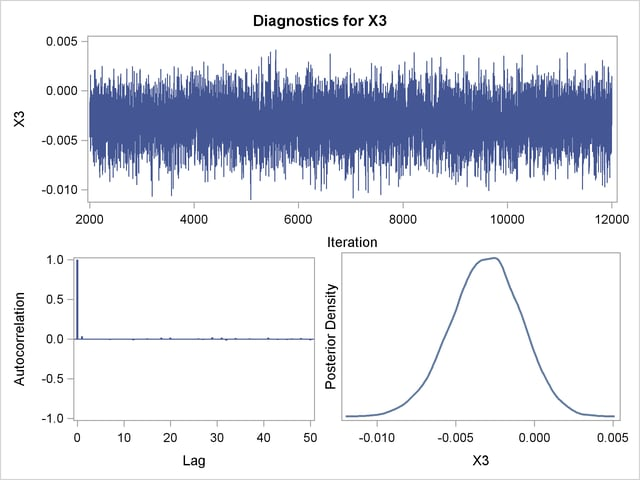Output 37.10.15 Diagnostic Plots for X4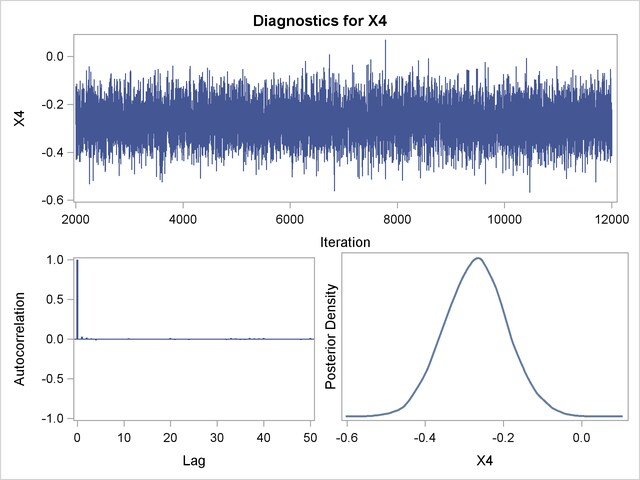Output 37.10.16 Diagnostic Plots for X5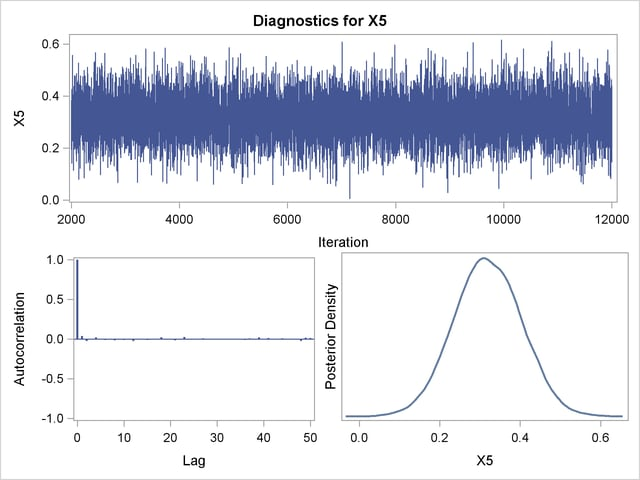Output 37.10.17 Diagnostic Plots for X6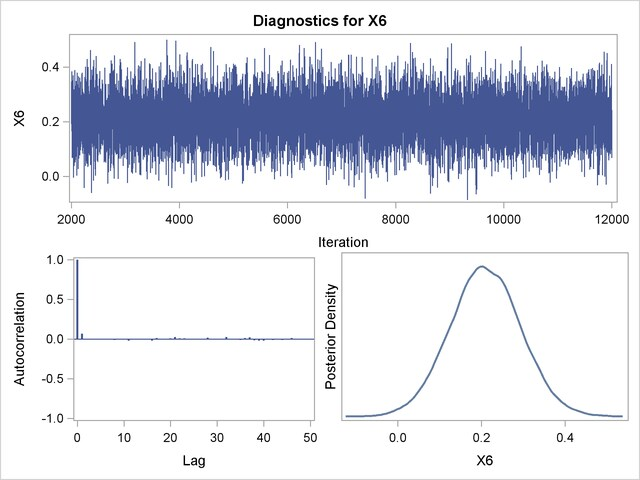In order to illustrate the use of an informative prior distribution, suppose that researchers expect that a unit increase in body mass index (X1) will be associated with an increase in the mean number of nodes of between 10% and 20%, and they want to incorporate this prior knowledge in the Bayesian analysis. For log-linear models, the mean and linear predictor are related by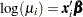. If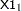and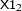are two values of body mass index,
and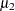are the two mean values, and all other covariates remain equal for the two values of X1, then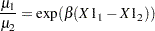so that for a unit change in X1,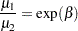If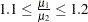, then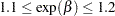, or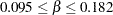. This gives you guidance in specifying a prior distribution for thefor body mass index. Taking the mean of the prior normal distribution to be the midrange of the values of, and taking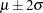to be the extremes of the range, an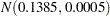is the resulting prior distribution. The second analysis uses this informative normal prior distribution for the coefficient of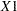and uses independent noninformative normal priors with zero means and variances equal tofor the remaining model regression parameters.

In the following BAYES statement, COEFFPRIOR=NORMAL(INPUT=NormalPrior) specifies the normal prior distribution for the regression coefficients with means and variances contained in the data set NormalPrior.

An analysis is performed using PROC GENMOD to obtain Bayesian estimates of the regression coefficients by using the following SAS statements:

```data NormalPrior;
input _type_ \$ Intercept X1-X6;
datalines;
Var  1e6   0.0005      1e6      1e6      1e6      1e6      1e6
Mean 0.0   0.1385      0.0      0.0      0.0      0.0      0.0
;
run;
```
```proc genmod data=Liver;
model Y = X1-X6 / dist=Poisson link=log;
bayes seed=1 plots=none coeffprior=normal(input=NormalPrior) ;
run;
ods graphics off;
```

The prior distributions for the regression parameters are shown in Output 37.10.18.

Output 37.10.18 Regression Coefficient Priors
The GENMOD Procedure

Bayesian Analysis

Independent Normal Prior for Regression
Coefficients
Parameter Mean Precision
Intercept 0 1E-6
X1 0.1385 2000
X2 0 1E-6
X3 0 1E-6
X4 0 1E-6
X5 0 1E-6
X6 0 1E-6

Initial values for the MCMC are shown in Output 37.10.19. The initial values of the covariates are joint estimates of their posterior modes. The prior distribution for X1 is informative, so the initial value of X1 is further from the MLE than the rest of the covariates. Initial values for the rest of the covariates are close to their MLEs, since noninformative prior distributions were specified for them.

Output 37.10.19 MCMC Initial Values and Seeds
Initial Values of the Chain
Chain Seed Intercept X1 X2 X3 X4 X5 X6
1 1 2.14282 0.010595 -0.01434 -0.00301 -0.28062 0.334983 0.231213

Goodness-of-fit, summary, and interval statistics are shown in Output 37.10.20. Except for X1, the statistics shown in Output 37.10.20 are very similar to the previous statistics for noninformative priors shown in Output 37.10.4 through Output 37.10.7. The point estimate for X1 is now positive. This is expected because the prior distribution on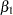is quite informative. The distribution reflects the belief that the coefficient is positive. Thedistribution places the majority of its probability density on positive values. As a result, the posterior density ofplaces more likelihood on positive values than in the noninformative case.

Output 37.10.20 Fit Statistics
Fit Statistics
DIC (smaller is better) 833.134
pD (effective number of parameters) 6.861

The GENMOD Procedure

Bayesian Analysis

Posterior Summaries
Parameter N Mean Standard
Deviation
Percentiles
25% 50% 75%
Intercept 10000 2.1393 0.2160 1.9929 2.1417 2.2845
X1 10000 0.0104 0.00685 0.00583 0.0106 0.0151
X2 10000 -0.0143 0.00236 -0.0159 -0.0143 -0.0127
X3 10000 -0.00318 0.00218 -0.00463 -0.00313 -0.00170
X4 10000 -0.2801 0.0798 -0.3342 -0.2807 -0.2258
X5 10000 0.3336 0.0834 0.2772 0.3337 0.3902
X6 10000 0.2333 0.0822 0.1791 0.2327 0.2892

Posterior Intervals
Parameter Alpha Equal-Tail Interval HPD Interval
Intercept 0.050 1.7161 2.5599 1.7075 2.5507
X1 0.050 -0.00323 0.0236 -0.00264 0.0241
X2 0.050 -0.0189 -0.00960 -0.0189 -0.00972
X3 0.050 -0.00754 0.00101 -0.00754 0.000963
X4 0.050 -0.4348 -0.1223 -0.4311 -0.1196
X5 0.050 0.1705 0.4970 0.1661 0.4915
X6 0.050 0.0696 0.3968 0.0655 0.3904Previous Page | Next Page | Top of Page